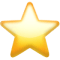Search app# Graphing Calculator - Math Pro

(0)
Price
Free
Category
Education & Utilities
Last Update
Jun 04, 2021
View in store

#### Store Performance Index

64%

Store Performance Index shows overall performance of your app on app stores. It is based on combined ASO & reviews metrics.

See more data

## Ratings & Reviews performance for Graphing Calculator - Math Pro

Ratings & Reviews performance provides an overview of what users think of your app. Here are the key metrics to help you identify how your app is rated by users and how successful is your review management strategy.

Number of reviews,
total

0

Avg rating,
total0.0

#### Description

2422 chars

Solve math problems, get help in doing homework, studying for tests, and learning math from basic arithmetic and algebra to more advanced topics, such as calculus, vector calculus, linear algebra, and differential equations. ⁃ Multi-functional scientific calculator ⁃ Mathematical formulas ⁃ Mathematical computations ⁃ Solutions to mathematical problems ⁃ 2D plots/graphs ⁃ History of computations (view and clear) ⁃ Sketchpad ⁃ Unit converter ⁃ Copy & paste ⁃ Beautiful themes Recommended by school teachers and university professors. Solve many types of mathematical problems - with more continually added. ⁃ Algebra •Fractions •Equation of a line •Quadratic formula •Factorization •Complete the square •Solve •Solve systems of equations •Roots of polynomials •Partial fraction decomposition ⁃ Vectors •Norm •Dot product •Cross product •Distance ⁃ Matrices •Matrix addition •Matrix multiplication •Determinant •Trace •Inverse matrix •Adjoint matrix •Matrix power •Matrix exponential •Eigenvalues & eigenvectors ‣exact ‣approximate ⁃ Complex numbers •Complex multiplication •Complex division •Real part •Imaginary part •Polar form •Rectangular form ⁃ Calculus •Derivative •Integral •Definite integral •Limit •Series •Taylor series ⁃ Vector calculus •Gradient •Divergence •Curl •Laplacian •Cartesian coordinates •Cylindrical polar coordinates •Spherical polar coordinates ⁃ Differential equations •Autonomous equations •Separable equations •Integrating factors •Second order linear ODEs •Linear systems •Check your answer ⁃ Statistics •Mean •Median •Mode •Standard deviation •Variance ⁃ Unit converter •Length •Weight •Volume •Area •Temperature •Time •Velocity •Angular velocity •Acceleration •Angular acceleration •Fuel consumption •Force •Pressure •Energy •Power •Binary •Angle •Density •Moment of inertia •Torque (moment of force) •Specific volume •Dynamic viscosity •Kinematic viscosity •Surface tension •Volume flux •Mass flux

User reviews affect conversion to installs and app rating. Featured and helpful reviews are the first to be noticed by users and in case of no response can affect download rate. This is why it is highly recommended to reply to them.#### About Graphing Calculator - Math Pro

Developed by Katarzyna Kowal.

Graphing Calculator - Math Pro is ranking in   Education & Utilities

Last update was at Jun 04, 2021and the current version is 1.1.

Graphing Calculator - Math Pro have a 0a user reviews.

Graphing Calculator - Math Pro was released in the App Store. It is developed by Katarzyna Kowal, who have also released the following apps.

Graphing Calculator - Math Pro has 0 user reviews.​

Graphing Calculator - Math Pro has an average rating of 0.0. ​The latest version of Graphing Calculator - Math Pro 1.1​ ​​was ​released on Jun 04, 2021​.